# interferometer

(redirected from interferometric)
Also found in: Dictionary, Thesaurus, Medical.

## interferometer:

see interferenceinterference,
in physics, the effect produced by the combination or superposition of two systems of waves, in which these waves reinforce, neutralize, or in other ways interfere with each other.
a computerized interferometer (see interference) that merges the images from two or more telescopes to obtain a single, large, enhanced image. The image in each telescope is made from electromagnetic waves (light waves from an optical telescope, radio waves
.

## interferometer

(in-ter-fĕ-rom -ĕ-ter) An instrument or system in which a beam of light or other radiation is split and subsequently recombined after the components of the split beam have traversed different pathlengths so that interference occurs and an interference pattern is produced. Interferometers use the effect of interference to measure some parameter – either the frequency components of the radiation undergoing interference, as with the Fabry–Perot interferometer or Fourier transform spectrometer, or spatial information about the radiation source, such as the apparent diameter of a star as with the Michelson stellar interferometer or the laser interferometers being used to detect gravitational waves.

The spatial interferometer also uses interference to make measurements but takes a different form. Radio, infrared, or optical waves collected by a group of separate telescopes observing the same object and linked to a common receiver are combined in order to improve the spatial resolution of the system: the resolving power is equivalent to that of a single instrument with an aperture equal to the largest distance separating the component telescopes. The interference pattern from a particular pair of telescopes records information on the relative amplitude and phase of the two beams being collected by that pair. The interference patterns from different pairs can then be combined and processed by computer to produce a highly detailed image of the source of the beams.

The radio interferometer is one of the two basic forms of radio telescope. Very great separations (baselines) may now be used (see VLBI; LBI). Technical problems have slowed the successful development of this type of interferometer to operate at optical and infrared wavelengths. New technology, however, especially adaptive optics, reduces the distorting effects of atmospheric turbulence, which is a serious problem for these optical and infrared interferometers. Since the end of the 1980s, optical interferometers, such as SUSI, have been used for the measurement of stellar diameters, the resolving of close binary stars, and wide-angle astrometry with milliarcsecond precision. Radio, IR, and optical interferometry carried out in space avoids the problems of atmospheric turbulence; see RadioAstron; VSOP.

## Interferometer

a measuring instrument that uses wave interference. Interferometers exist for sound and electromagnetic waves, such as optical waves (ultraviolet, visible, and infrared spectral regions) and radio waves of various lengths. Interferometers are very widely used. Acoustic and radio interferometers are used to measure the propagation velocity of radio and acoustic waves, as well as the distances between two emitters of waves or between an emitter and a reflector (that is, they are used as range finders). Optical interferometers, which are most commonly used, will be described below. They are used to measure the wavelengths of spectral lines, the indexes of refraction of transparent mediums, absolute and relative distances, and the angular dimensions of stars, as well as to monitor the quality of optical components and their surfaces and the finishing quality of metal surfaces.

The principle of operation of all interferometers is the same; the instruments differ only in the method of generation of coherent waves and in the parameter that is measured directly. A beam of light is spatially separated by a device into two or more coherent beams, which traverse different optical paths and then converge. An interference pattern, whose shape (the form and relative location of the interference maximums and minimums), depends on the mode of separation of the light beam into coherent beams, the number of interfering beams, their path differences, the relative intensity, the dimensions of the source, and the spectral composition of the light, is observed at the point of convergence.

The methods for generating coherent beams in interferometers vary greatly; therefore, there is a large number of interferometer designs. Depending on the number of interfering light beams, interferometers may be divided into multiple-beam and two-beam types.

The Michelson interferometer (see Figure 1) is an example of a two-beam interferometer. The parallel beam of light from the source L is divided into beams 1 and 2 by the semitransparent plate P1. Upon reflection from the mirrors M1 and M2 and after passing through the plate P1 for a second time, both beams pass through the objective 02 and interfere in its focal plane D. The optical path difference is Δ = 2(AC – AB) = 21, where l is the distance between the mirror M2 and the virtual image M1 of the mirror M1 in the plate P1. Thus, the observed interference pattern is equivalent to interference in a layer of air of thickness l. If the mirror Mi is located in such a manner that M1 and M2 are parallel, then equal-inclination fringes are formed; they are localized in the focal plane of the objective 02 and are concentric rings. However, if M2 and M1 form an air wedge, equal-thickness fringes form that are localized in the plane of the wedge M2M1 and are parallel lines.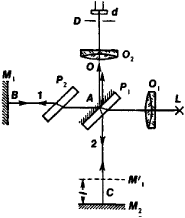Figure 1. Diagram of a Michel-son interferometer: P2 is a plate that compensates for the additional path difference that arises because ray 1 passes through plate P1, twice

The Michelson interferometer is widely used in physical measurements and engineering instruments. It was used to determine the absolute wavelength of light for the first time and to prove the independence of the speed of light from the earth’s motion. Displacement of one of the mirrors in the Michelson interferometer makes possible continuous variation of Δ, whereas the relationship between the intensity of the central spot and Δ makes possible, in turn, analysis of the spectral composition of the incident radiation with a resolution of 1/Δ cm1. This is the design principle of the Fourier spectrometers, which are used in the long-wave infrared region (50–1000 microns [μ]) to solve problems in solid-state physics, organic chemistry, polymer chemistry, and plasma studies. A resolution of ∼0.005 cm1 in the wavelength band 0.8–3.5 μ was achieved using a Fourier spectrometer, in which the path difference was controlled and measured with a helium-neon laser.

The combination of a Michelson interferometer with a prism monochromator (Figure 2, a)—a Kösters interference comparator—is used for absolute and relative measurement of the length of end bars (end blocks) by comparison either with the wavelength of light or with each other, with a precision of ≈0.025 μ,. The combination of this device with a laser (with the frequency stabilization of ∼ 2 X 10−9) makes possible measurement of lengths of the order of 10 m with the same absolute precision. The substitution of reflecting trihedrals for the flat mirrors in a Michelson interferometer makes it possible to use the instrument for the measurement of angles with a precision of up to 10−6 radians. The combination of a Michelson interferometer with a microscope (microinterferometer of V. P. Lin-nik) makes possible the determination of the magnitude and shape of the microroughness of metallic surfaces from the interference pattern.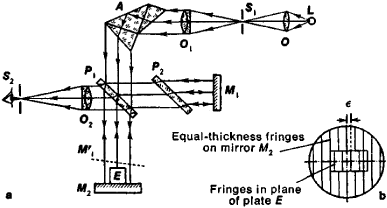Figure 2. (a) Diagram of a Kosters interferometer (symbols are the same as for the Michelson interferometer):(A)dispersing prism, (£) end bar, (S, ) monochromator slit; (b) interference pattern

Two-beam interferometers designed for the determination of the indexes of refraction of gases and liquids are called interferential refractometers; one such device was designed by J. Jamin (Figure 3). A light beam S, after reflection from the front and rear surfaces of the plate P1, is divided into two beams S1 and S2. After passing through the cells C1 and C2, the beams reflected by the surfaces of the plate P2 enter the telescope T, where they interfere, forming equal-inclination fringes. If one of the cells is filled with a material with index of refraction n1 and the other is filled with a material with index of refraction n2, the displacement of the interference pattern by a number of fringes m, as compared to the case when both cells are filled with the same material, yields Δ n = n1n2 = mλ/l, where l is the length of the cell.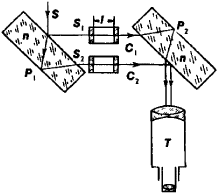Figure 3. Diagram of a Jamin interferometer

Variants of the Jamin interferometer are the Mach-Zehnder and Rozhdestvenskii interferometers (Figure 4), in which two semitransparent plates P1 and P2 and two mirrors M1 and M2 are used. The distance between the beams S1 and S2 may be made very large in these interferometers, which facilitates the mounting of various objects of study in one of the beams. For this reason, these instruments are widely used in the studies of air and gas dynamics.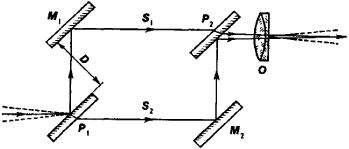Figure 4. Diagram of a Rozhdestvenskii interferometer

In the Rayleigh interferometer (Figure 5), the interfering beams are separated by two slit diaphragms D. After passing through the cells C1 and C2, the beams are collected in the focal plane by the objective 02, where the interference pattern consisting of equal-inclination fringes forms; it is viewed through the eye-piece O3. In this case, parts of the beams emerging from the diaphragms pass below the cells and form an interference pattern of their own, which is located below the first pattern. A difference between the indexes of refraction n1 and n2 of the materials in the cells leads to a displacement of the upper pattern with respect to the lower. The quantity Δ n may be determined from the magnitude of displacement in terms of the number of fringes m.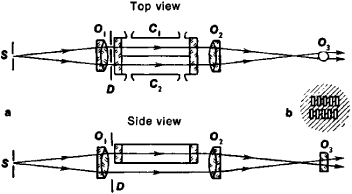Figure 5. (a) Diagram of a Rayleigh interferometer, (b) interference pattern

The precision of measurement of the index of refraction with interferential refractometers is very high (up to seven or even eight decimal places).

The Michelson stellar interferometer (Figure 6) is used to measure the angular dimensions of stars and the angular separations between binary stars. The light from a star is reflected by the mirrors M1, M2, M3, and M4 and forms an interference pattern in the focal plane of the telescope. The angular separation between adjacent maximums is θ = λ/D (Figure 6, b). When two stars are located close to each other at an angular separation ϕ), two interference patterns are observed in the telescope, likewise displaced by the angle ϕ. D is varied to achieve the least resolution of the pattern, which will be the case for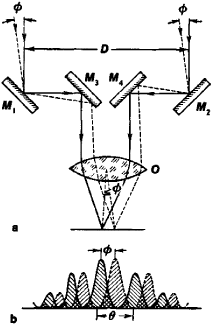Figure 6. (a) Diagram of a Michelson stellar interferometer, (b) interference patterns

ϕ = 1/2θ = λ/2D. The quantity ϕ may be calculated from this relationship.

The multiple-beam Fabry-Perot interferometer (Figure 7) consists of two glass or quartz plates P1 and P2 whose facing surfaces are covered by highly reflective (85–98 percent) coatings. As a result of multiple reflection from the mirrors, a parallel beam of light incident from the objective O1 forms a large number of parallel coherent beams with a constant path difference between adjacent beams. The multiple-beam interference in the focal plane L of the objective O2 generates an interference pattern in the form of concentric rings with sharp and intense maximums, whose location depends on the wavelength. For this reason, the Fabry-Perot interferometer leads to the dispersion of complex radiation into a spectrum. The Fabry-Perot interferometer is used as a high-resolution interferential spectral instrument. Special scanning Fabry-Perot interferometers with photoelectric recording are used for spectral studies in the visible, infrared, and centimeter wavelength bands. The optical resonators of lasers whose active medium is located between the mirrors of an interferometer are special cases of a Fabry-Perot interferometer.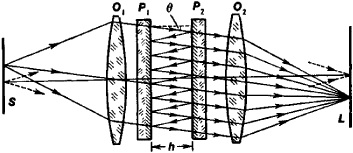Figure 7. Diagram of a Fabry-Perot interferometer (S is the light source)

The multiple-beam interferometers also include various diffraction gratings, which are used as interferential spectral instruments.

### REFERENCES

Landsberg, G. S. Optika, 4th ed. Moscow, 1957. (Obshchii kurs fiziki, vol. 3.)
Zakhar’evskii, A. N. Interferometry. Moscow, 1952.
Korolev, F. A. Spektroskopiia vysokoi razreshaiushchei sily. Moscow, 1953.
Tolansky, S. Spektroskopiia vysokoi razreshaiushchei sily. Moscow, 1955. (Translated from English.)
Infrakrasnaia spektroskopiia vysokogo razresheniia. Moscow, 1972. (Translated from French.)
Zhakino, P. “Poslednie dostizheniia interferentsionnoi spektroskopii.” Uspekhi fizicheskikh nauk. 1962, vol. 78, p. 123.

V. I. MALYSHEV

## interferometer

[‚in·tə·fə′räm·əd·ər]
(optics)
An instrument in which light from a source is split into two or more beams, which are subsequently reunited after traveling over different paths and display interference.

## interferometer

An instrument that measures the wavelengths of light and distances. It transmits two radio signals or beams of light and uses the interference principle (how they reinforce or neutralize each other) to determine the measurement.
References in periodicals archive ?
Zazula, "Heartbeat and respiration detection from optical interferometric signals by using a multimethod approach," IEEE Transactions on Biomedical Engineering, vol.
Figure 8 shows the intensity signal [I.sub.y](k) of the seismic wave and the signal in a time-frequency plane measured by the interferometric seismometer.
By analyzing the statistical and signal properties of interferometric phase, this paper proposes the concept of nonlinear phase model and studies the phase filtering and unwrapping method based on the model.
In accordance with the principle of superposition, an interferometric electric field vector [E.sub.0], at a point on the photodetector, is given by
 and can be decomposed into sums of intensity, polarimetric and interferometric contributions.
The interferometric data channels on the SSAMII can be used to generate bathymetric estimates that are co-registered with the output SAS images.
The interferometric radar in this configuration provides a two-dimensional complex radar cross-section (RCS) images, with amplitude and phase information.
In Section 2, the interferometric coherence is presented.
Standard electronic techniques are adequate for processing ultrasonic echoes that travel at the speed of sound, interferometric techniques are required to extract the reflected optical signals from the infrared light used in OCT.
Selim Unlu from Boston University's Electrical and Computer Engineering Department has developed the "Interferometric Reflectance Imaging Sensor" (IRIS) which can detect multiple viruses in a single blood sample instead of running numerous expensive and time-consuming tests to determine each disease-causing virus found in the blood.
ALMA is a large interferometric set of telescopes somewhat similar in concept to the SKA but working at much shorter wavelengths and designed to answer quite different scientific problems.
Heat transfer by natural convection of the air on vertical plates and in mortises was investigated on a model by the interferometric method.

Site: Follow: Share:
Open / Close